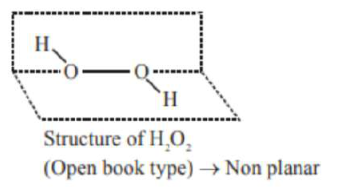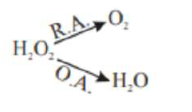Question:

The correct statements about $\mathrm{H}_{2} \mathrm{O}_{2}$ are:

(A) used in the treatment of effluents.

(B) used as both oxidising and reducing agents.

(C) the two hydroxyl groups lie in the same plane.

(D) miscible with water.

Choose the correct answer from the options given below:

1. $(\mathrm{A}),(\mathrm{B}),(\mathrm{C})$ and $(\mathrm{D})$

2. (A), (B) and (D) only

3. $(\mathrm{B}),(\mathrm{C})$ and $(\mathrm{D})$ only

4.  $(\mathrm{A}),(\mathrm{C})$ and $(\mathrm{D})$ only

Correct Option: , 2

Solution:$\mathrm{H}_{2} \mathrm{O}_{2}$ is used in the treatment of effluents.act as both O.A $\backslash \&$ R.A.

$\mathrm{H}_{2} \mathrm{O}_{2}$ is miscible in water due to hydrogen bonding.